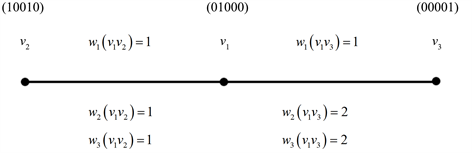# 在占空比无线传感器网络中寻找多约束路径的算法Algorithms for Finding Multi-Constrained Paths in Duty-Cycle Wireless Sensor Networks

DOI: 10.12677/AAM.2021.104144, PDF, HTML, XML, 下载: 7  浏览: 26  科研立项经费支持

Abstract: The duty-cycle wireless sensor network has been widely used in the intrusion detection, monitoring of seismic activity and some remote areas. After an event occurs, the delay, energy consumption and number of hops of data transmission should be constrained. Based on this requirement, in this paper, we deal with the NP-complete problem: multi-constrained path problem in the duty-cycle wireless sensor network (DC-WSN). We will propose a polynomial time algorithm to design a source-to-sink data delivery rout under multi-stringent constraints.

1. 引言

1) 研究了具有一般拓扑结构的DC-WSNs中的多约束路径   问题。此外，在本文同时考虑的各种约束中，任意一对节点之间的传输延迟可以与DC-WSNs的时间扩展图  的构造相结合。

2) 重点讨论了在时间展开图中选择的路径与原始网络中所需要的非循环路径之间的关系。

2. 术语与网络模型

1) ${u}_{i}$${u}_{i+1}$ 是相邻节点；

2) 对于 $W$ 中的每个边 ${u}_{i}{u}_{i+1}$，数据包可以在 ${t}_{i}$ 时间之前成功地从 ${u}_{i}$ 传送到 ${t}_{i+1}$，在 ${t}_{i+1}$ 时间被 ${u}_{i+1}$ 接收。

DC-WSN中的多约束路径问题(WSN)表示为DC-MCP $\left(G,s,t,{w}_{1},{w}_{2},{w}_{3},{c}_{1},{c}_{2},{c}_{3}\right)$ (MCP $\left(G,s,t,{w}_{1},{w}_{2},{w}_{3},{c}_{1},{c}_{2},{c}_{3}\right)$ )，为求出一条从 $s$$t$ 的可行路径 $P$，使得 ${W}_{1}\left(P\right)\le {c}_{1}$ (即 ${w}_{1}\le {c}_{1}$ )， ${w}_{2}\left(P\right)\le {c}_{2}$${w}_{3}\left(P\right)\le {c}_{3}$。如果存在这样的路径，则该路径是DC-MCP $\left(G,s,t,{w}_{1},{w}_{2},{w}_{3},{c}_{1},{c}_{2},{c}_{3}\right)$ (MCP $\left(G,s,t,{w}_{1},{w}_{2},{w}_{3},{c}_{1},{c}_{2},{c}_{3}\right)$ )。然后，本文的主要问题是找到DC-MCP $\left(G,s,t,{w}_{1},{w}_{2},{w}_{3},{c}_{1},{c}_{2},{c}_{3}\right)$ 的解。接下来，我们将分两步提出一个针对这个问题的多项式时间算法。

2.1. 具有三个权值函数的时间扩展图

1) 设 $d=⌈{c}_{1}⌉$${G}_{1}$ 的点集为 $V\left({G}_{1}\right)=\left\{{v}_{i}\left[t\right]:\text{\hspace{0.17em}}{v}_{i}\in V,\text{\hspace{0.17em}}1\le t\le d\right\}\cup \left\{{t}_{0}\left[0\right]\right\}$

2) ${G}_{1}$ 的有向边集为

$\begin{array}{l}E\left({G}_{1}\right)=\left\{\left(t\left[i\right],{t}_{0}\left[0\right]\right):\text{\hspace{0.17em}}1\le i\le d\right\}\text{\hspace{0.17em}}\cup \left\{\left({v}_{i}\left[x\right],{v}_{j}\left[y\right]\right):\text{\hspace{0.17em}}{v}_{i}{v}_{j}\in E\left(G\right),\text{\hspace{0.17em}}1\le x

3) 定义两个权值函数： ${w}^{j}:E\to {R}^{+}$$j=2,3$，如下所示： ${w}^{2}\left(\left(t\left[i\right],{t}_{0}\left[0\right]\right)\right)={w}^{3}\left(\left(t\left[i\right],{t}_{0}\left[0\right]\right)\right)=0$。对于任何的 $\left({v}_{i}\left[t\right],{v}_{j}\left[{t}^{\prime }\right]\right)\in E\left({G}_{1}-{t}_{0}\left[0\right]\right)$${w}^{j}\left(\left({v}_{i}\left[t\right],{v}_{j}\left[{t}^{\prime }\right]\right)\right)={w}_{j}\left({v}_{i}{v}_{j}\right)$ 对于 $j=2,3$

${{G}^{\prime }}_{1}={G}_{1}-{t}_{0}\left[0\right]$${N}_{{G}^{\prime }}^{+}\left(v\right)=\left\{u:\left(v,u\right)\in E\left({G}^{\prime }\right)\right\}$，其中 $v\in V\left({G}^{\prime }\right)$。那么以下两个观察结果是显而易见的。

2.2. 创建一个新的权值函数

3. 多项式时间路由算法Figure 1. An undirected graph $G=\left(V\left(G\right),E\left(G\right)\right)$ with three weight functions w1, w2 and w3, where $V\left(G\right)=\left\{{v}_{1},{v}_{2},{v}_{3}\right\}$ and $E\left(G\right)=\left\{{v}_{1}{v}_{2},{v}_{1}{v}_{3}\right\}$

$|V\left(G\right)|=n$$|E\left(G\right)|=m$$|V\left({{G}^{\prime }}_{1}\right)|=dn$$|Q|=\left(x+1\right)|V\left({{G}^{\prime }}_{1}\right)|=\left(x+1\right)dn$。因为 $V\left(T\right)\subseteq V\left({{G}^{\prime }}_{1}\right)$，步骤2和步骤5需要 $O\left(2|V\left({{G}^{\prime }}_{1}\right)|\right)$ 时间复杂度。步骤3~4的时间复杂度是 $O\left(2|Q|\right)$。松弛算法需要 $O\left(1\right)$ 时间。根据观察2.3，for循环(11~12行)最多有 $|{N}_{{{G}^{\prime }}_{1}}^{+}\left({v}_{i}\left[t\right]\right)|\le |{N}_{G}\left({v}_{i}\right)|\le |E\left(G\right)|$ 次迭代，所以步骤11~12的时间复杂度是 $O\left(m\right)$。在2~13行中，每次执行if循环， $E\left({{G}^{\prime }}_{1}\right)\E\left(T\right)$ 中的一条边将被加到集合 $T$ 中。最多有 $|E\left(T\right)|=|V\left(T\right)|-1<|V\left({{G}^{\prime }}_{1}\right)|$ 次迭代。所以，步骤2~13需要 $O\left(dn\left(2dn+2\left(x+1\right)dn+m\right)\right)=O\left(x{c}_{1}^{2}{n}^{2}+{c}_{1}mn\right)$ 时间。步骤15的时间复杂度为 $O\left(xd\right)$。因为 $\stackrel{˜}{{P}^{\prime }}$ 是图 $G$ 中的路(见定理3.1)，所以步骤16需要 $O\left(n\right)$ 次。因此，总时间复杂度为 $O\left(x{c}_{1}^{2}{n}^{2}+{c}_{1}mn\right)=O\left(x{c}_{1}^{2}{n}^{2}+{c}_{1}{n}^{3}\right)$

${w}^{2}\left({P}_{3}\right)={w}^{2}\left(P\left[s\left[1\right],u\left[{t}_{1}\right]\right]\right)+{w}^{2}\left({P}_{2}\left({t}_{1}\right)\right)={w}^{2}\left(P\left[s\left[1\right],u\left[{t}_{1}\right]\right]\right)+{w}^{2}\left({P}_{1}\right)<{w}^{2}\left(P\right)\le {c}_{2}$ . (3.1)

$w\left({P}_{3}\right) . (3.2)

Since $\left({u}_{i}\left[{t}_{7}\right],{u}_{i+1}\left[{t}_{8}\right]\right)\in E\left({P}_{1}\right),$ $⌈{w}_{1}\left({u}_{i}{u}_{i+1}\right)⌉\le {t}_{8}-{t}_{7}$，故产生矛盾。

4. 结论

NOTES

*通讯作者。

  Gu, Y. and He, T. (2011) Dynamic Switching-Based Data Forwarding for Low-Duty-Cycle Wireless Sensor Networks. IEEE Transactions on Mobile Computing, 10, 1741-1754. https://doi.org/10.1109/TMC.2010.266  Guo, S., Jose, S., He, L., Gu, Y. and Jiang, B. (2014) Opportunistic Flooding in Low-Duty-Cycle Wireless Sensor Networks with Unreliable Links. IEEE Transactions on Computers, 63, 2787-2802. https://doi.org/10.1109/TC.2013.142  Li, Z., Peng, Y., Qiao, D. and Zhang, W. (2012) LBA: Lifetime Balanced Data Aggregation in Low Duty Cycle Sensor Networks. 2012 Proceedings IEEE INFOCOM, Orlando, FL, 25-30 March 2012, 1844-1852. https://doi.org/10.1109/INFCOM.2012.6195559  Ye, W., Heidemann, J. and Estrin, D. (2002) An Energy-Efficient MAC Protocol for Wireless Sensor Networks. Twenty-First Annual Joint Conference of the IEEE Computer and Communications Societies, New York, 23-27 June 2002, 1567-1576.  Yoo, H., Shim, M. and Kim, D. (2012) Dynamic Duty-Cycle Scheduling Schemes for Energy-Har Vesting Wireless Sensor Networks. IEEE Communications Letters, 16, 202-204. https://doi.org/10.1109/LCOMM.2011.120211.111501  Kim, J., Lin, X., Shroff, N.B. and Sinha, P. (2010) Minimizing Delay and Maximizing Lifetime for Wireless Sensor Networks with Anycast. IEEE/ACM Transactions on Networking, 18, 515-528. https://doi.org/10.1109/TNET.2009.2032294  Naveen, K.P. and Kumar, A. (2010) Tunable Locally-Optimal Geographical Forwarding in Wireless Sensor Networks with Sleep-Wake Cycling Nodes. 2010 Proceedings IEEE INFOCOM, San Diego, CA, 14-19 March 2010, 1-9. https://doi.org/10.1109/INFCOM.2010.5461956  Su, L., Ding, B., Yang, Y., Abdelzaher, T.F., Cao, G. and Hou, J.C. (2009) oCast: Optimal Multicast Routing Protocol for Wireless Sensor Networks. 2009 17th IEEE International Conference on Network Protocols, Plainsboro, NJ, 13-16 October 2009, 151-160. https://doi.org/10.1109/ICNP.2009.5339689  Chen, S. and Nahrstedt, K. (1998) On Finding Multi-Constrained Paths. 1998 IEEE International Conference on Communications. Conference Record. Affiliated with SUPERCOMM’98 (Cat. No.98CH36220), Atlanta, GA, 7-11 June 1998, 874-879.  Jaffe, J.M. (1984) Algorithms for Finding Paths with Multiple Constraints. Networks, 14, 95-116. https://doi.org/10.1002/net.3230140109  Bondy, J.A. and Murty, U.S.R. (2007) Graph Theory. Springer, New York. https://doi.org/10.1007/978-1-84628-970-5